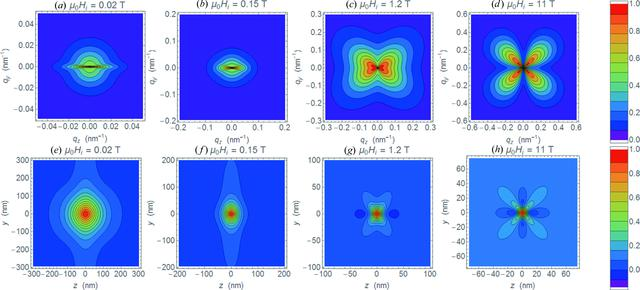disable zoom     view article Figure 11 (a)–(d) Contour plots of normalized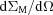[equation (6)] at applied magnetic fields as indicated (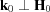;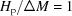;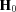is horizontal). For h2(qR) and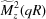, we used the form factor of a sphere with a radius of R = 5 nm [equation (44); S(q) = 1]. (e)–(h) Corresponding two-dimensional correlation functions c(y, z), which were computed according to equation (37)().JOURNAL OFAPPLIEDCRYSTALLOGRAPHY
ISSN: 1600-5767
Volume 48| Part 5| October 2015| Pages 1437-1450